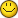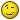Boll Squeeze with JMA for MT4 - page 21389

maumax:
How to use is found in the photos, also attached is the dayimpluse indicator, I find it great for making sure you enter the correct direction for the day, just drag a 34 ema into the window with the indicator

If BBsqueeze is red, what does that mean? How does one short the currency, is it that the impulse must cross down into the EMA, and BBSqueeze is red? Does white in BBSqueeze mean its consolidating? How did you get the envelope around BBSqueeze, and what settings?

Thanks,

ET23

so Hello to everybody

I am looking for an indicator which works in separate window-BB+CCI.

Have anybody seen it?...and look for Trend Oscillator as well..

Iam working on channel method....thanks for help

luky4

Hi: I am new bee here and not a programmer. I want to get started in Swing Trading in FOREX keeping my Day Job....any input1853

Start by educating yourself first. Forex is a very risky business. Start learning the basic. You will find various helpful sites and forum for that. Give it the needed time. Dont hurry up. Check my sig.

avrint:
Hi: I am new bee here and not a programmer. I want to get started in Swing Trading in FOREX keeping my Day Job....any input4

Thanks for your help......3

maumax:
Here is the Squeeze indicator by Nick Bilak, I found the indicator works better for me with the following settings extern int bolPrd=20;extern double bolDev=2.0;extern int keltPrd=18;extern double keltFactor=1.7;//1.5extern int momPrd=14; Put the bbsqueeze on your chart, then add the indicator from the previous post in the same window.This squeeze indicator has already been posted in text form, in this thread

Hello Maumax and all,

Thank you for posting the different helping tools with the Bol Squeeze indicator! It has been very helpful to my tradings because among the many indicators, I have found that the Bol Squeeze is one of the most reliable signals for an impending move, in any markets, any timeframes one trades. For this reason, I think it'd be most useful if one can be alerted whenever a squeeze occurs. Since I'm a novice in using MT4, I wonder any of our friends here can help to create an alert feature into Mr. Nick Bilak's squeeze indicator? His original code for the indicator is as follows:

#property indicator_separate_window

#property indicator_buffers 6

#property indicator_color1 Green

#property indicator_color2 Blue

#property indicator_color3 LimeGreen

#property indicator_color4 RoyalBlue

#property indicator_color5 Red

#property indicator_color6 White

//---- input parameters

extern int bolPrd=20;

extern double bolDev=2.0;

extern int keltPrd=20;

extern double keltFactor=1.5;

extern int momPrd=12;

//---- buffers

double upB[];

double loB[];

double upK[];

double loK[];

double upB2[];

double loB2[];

double mm[];

int i,j,slippage=3;

double breakpoint=0.0;

double ema=0.0;

int peakf=0;

int peaks=0;

int valleyf=0;

int valleys=0, limit=0;

double ccis,ccif;

double delta=0;

double ugol=0;

int init()

{

//---- indicators

IndicatorBuffers(7);

SetIndexStyle(0,DRAW_HISTOGRAM,STYLE_SOLID,2);

SetIndexBuffer(0,upB);

SetIndexEmptyValue(0,EMPTY_VALUE);

SetIndexStyle(1,DRAW_HISTOGRAM,STYLE_SOLID,2);

SetIndexBuffer(1,loB);

SetIndexEmptyValue(1,EMPTY_VALUE);

SetIndexStyle(2,DRAW_HISTOGRAM,STYLE_SOLID,2);

SetIndexBuffer(2,upB2);

SetIndexEmptyValue(2,EMPTY_VALUE);

SetIndexStyle(3,DRAW_HISTOGRAM,STYLE_SOLID,2);

SetIndexBuffer(3,loB2);

SetIndexEmptyValue(3,EMPTY_VALUE);

SetIndexBuffer(6,mm);

SetIndexStyle(4,DRAW_ARROW);

SetIndexBuffer(4,upK);

SetIndexEmptyValue(4,EMPTY_VALUE);

SetIndexArrow(4,167);

SetIndexStyle(5,DRAW_ARROW);

SetIndexBuffer(5,loK);

SetIndexEmptyValue(5,EMPTY_VALUE);

SetIndexArrow(5,167);

return(0);

}

int start()

{

int counted_bars=IndicatorCounted();

int shift,limit;

double diff,d,std,bbs;

if (counted_bars<0) return(-1);

if (counted_bars>0) counted_bars--;

limit=Bars-31;

if(counted_bars>=31) limit=Bars-counted_bars-1;

for (shift=limit;shift>=0;shift--) {

//d=iMomentum(NULL,0,momPrd,PRICE_CLOSE,shift);

d=LinearRegressionValue(momPrd,shift);

mm[shift]=d;

if(d>0) {

if (mm[shift]>mm[shift+1]) {

upB[shift]=d;

upB2[shift]=0;

loB[shift]=0;

loB2[shift]=0;

} else {

upB[shift]=0;

upB2[shift]=d;

loB[shift]=0;

loB2[shift]=0;

}

} else {

if (mm[shift]<mm[shift+1]) {

loB[shift]=d;

loB2[shift]=0;

upB[shift]=0;

upB2[shift]=0;

} else {

loB[shift]=0;

loB2[shift]=d;

upB[shift]=0;

upB2[shift]=0;

}

}

diff = iATR(NULL,0,keltPrd,shift)*keltFactor;

std = iStdDev(NULL,0,bolPrd,MODE_SMA,0,PRICE_CLOSE,shift);

bbs = bolDev * std / diff;

if(bbs<1) {

upK[shift]=0;

loK[shift]=EMPTY_VALUE;

} else {

loK[shift]=0;

upK[shift]=EMPTY_VALUE;

}

}

return(0);

}

//+------------------------------------------------------------------+

double LinearRegressionValue(int Len,int shift) {

double SumBars = 0;

double SumSqrBars = 0;

double SumY = 0;

double Sum1 = 0;

double Sum2 = 0;

double Slope = 0;

SumBars = Len * (Len-1) * 0.5;

SumSqrBars = (Len - 1) * Len * (2 * Len - 1)/6;

for (int x=0; x<=Len-1;x++) {

double HH = Low[x+shift];

double LL = High[x+shift];

for (int y=x; y<=(x+Len)-1; y++) {

HH = MathMax(HH, High[y+shift]);

LL = MathMin(LL, Low[y+shift]);

}

Sum1 += x* (Close[x+shift]-((HH+LL)/2 + iMA(NULL,0,Len,0,MODE_EMA,PRICE_CLOSE,x+shift))/2);

SumY += (Close[x+shift]-((HH+LL)/2 + iMA(NULL,0,Len,0,MODE_EMA,PRICE_CLOSE,x+shift))/2);

}

Sum2 = SumBars * SumY;

double Num1 = Len * Sum1 - Sum2;

double Num2 = SumBars * SumBars-Len * SumSqrBars;

if (Num2 != 0.0) {

Slope = Num1/Num2;

} else {

Slope = 0;

}

double Intercept = (SumY - Slope*SumBars) /Len;

//debugPrintln(Intercept+" : "+Slope);

double LinearRegValue = Intercept+Slope * (Len - 1);

return (LinearRegValue);

}

Many thanks!4

Hello every one. I m from france. I like markets. And i would like to make some trading...I'v done it 10 years ago on stocks markets and options.

Now i would like to try on the forex...

See you..

Thomas4

Make your pardon, i think my precedent post was not on the good localisation...in the site

Anyaway, i have a question : i m interested in the BBsqueeze from Carter.

But, why do we have a lot of versions ? I thought the only variations were the parameters of the indicators...

Any idea to answer me ? Thanks and kisses from France37

Squeeze is a great signal.. I have traded it for some time with great results.

THANXXXX102

After loosing money in a few forex live trading, I am so desperate to look for the best buy and sell indicator. Most Buy and sell indicator that I got are not really helpful as buy/sell indictor is still appeared even the when the pairs have been overbought or oversold.

Anyone out there that can help me..pls..